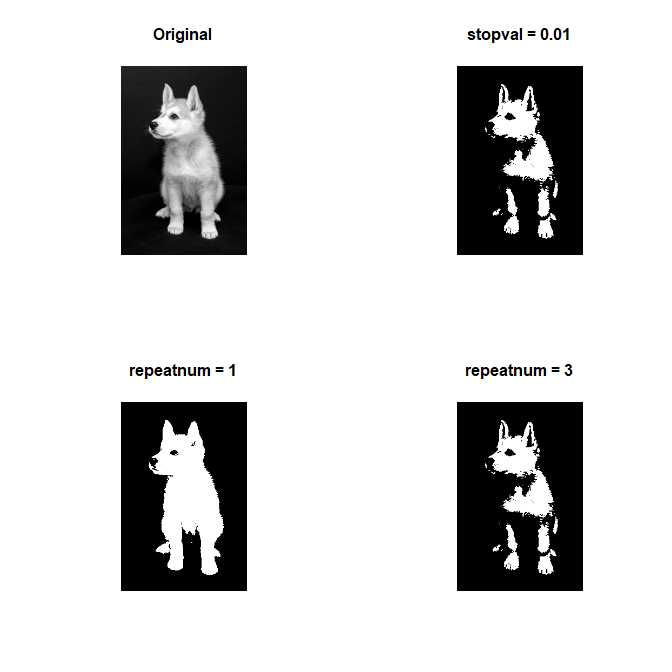# Getting Started with imagerExtara

#### 2018-07-03

imagerExtra provides several advanced functions for image processing.

I will introduce the functions briefly.

imagerExtara is based on imager.

See the vignettes of imager if you aren’t familiar with imager.

# Preparation

Most of the functions of imagerExtra are for grayscale image.

See the vignette Treating Color Image with imagerExtra if you want to treat color image with imagerExtra

Let’s prepare a grayscale image.

If you want to treat color image, see the vignette “treating color image with imagerExtra”.

library(imagerExtra)
g <- grayscale(boats)
plot(g)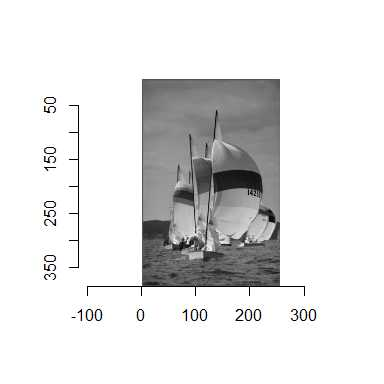# Piecewise Affine Histogram Equalization

Piecewise Affine Equalization (PAE) enhances contrast of image.

PAE has three parameters: N, smin, and smax.

However, we should not change smin. See Jose-Luis Lisani, et al., IPOL, 2 (2012), pp. 243-265. for detail.

That’s why PAE has two parameters in practice.

## the parameter N

N controls how the input gray level will be mapped in the output image.

We don’t have a priori choice for N. You will tune N mainly.

PAE acts as Histogram Equalization if N is large.

layout(matrix(1:4, 2, 2))
plot(g, main = "Original")
plot(EqualizePiecewise(g, 2), main = "N = 2")
plot(EqualizePiecewise(g, 10), main = "N = 10")
plot(EqualizePiecewise(g, 1000), main = "N = 1000")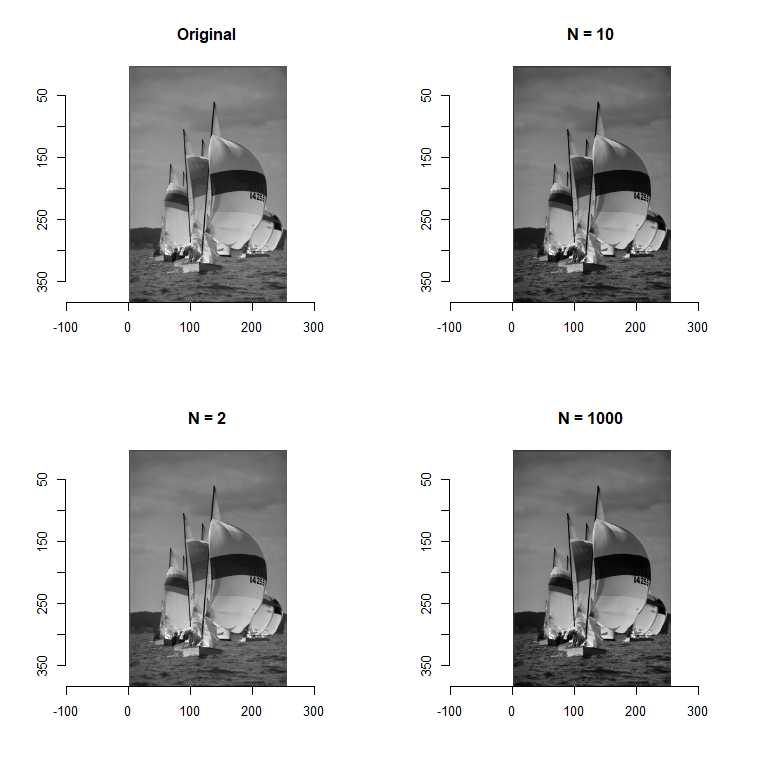## the parameter smax

smax controls the upper limit of contrast stretching.

If you want to prevent excessive enhancement of contrast, you should make smax low.

# Simplest Color Balance

Simplest Color Balance (SCB) enhances contrast of image.

SCB assumes that objectives in image have moderate pixel values.

That’s why SCB saturates a percentage sleft % of the pixels on the left side of the histogram, and a percentage sright % of the pixels on the right side of the histogram.

See Nicolas Limare, et al., IPOL, 1 (2011), pp. 297-315. for detail.

## the parameters sleft and sright

sleft is a saturation percentage of the pixels on the left side of the histogram.

sright is a saturation percentage of the pixels on the right side of the histogram.

## the parameter range

SCB applies affine transformation after saturating pixels.

range defines the output range.

Default of range is [0,255]. You may prefer [0,1].

layout(matrix(1:2, 1, 2))
plot(g, main = "Original")
plot(BalanceSimplest(g, 1, 1), main = "sleft = 1, sright = 1")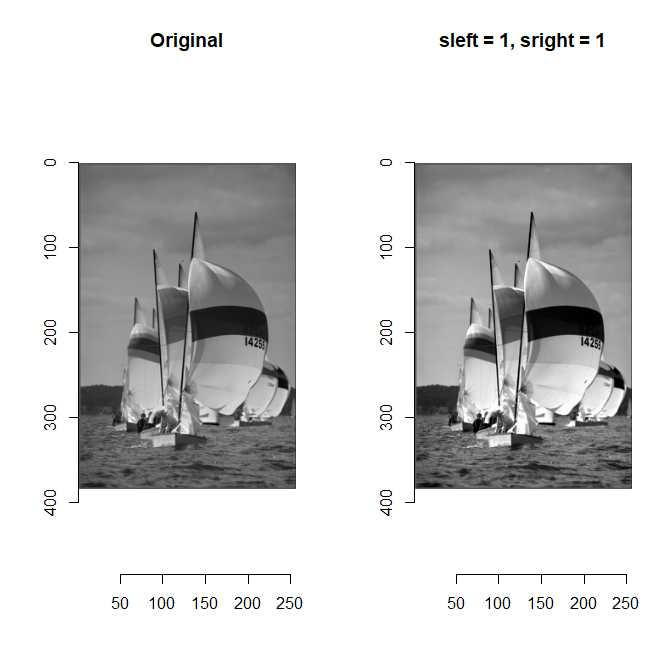# Screened Poisson Equation

Screend Poisson Equalization (SPE) enhances contrast of image.

The distinction of SPE is that SPE corrects the inhomogeneous background of image.

See Jean-Michel Morel, et al., IPOL, 4 (2014), pp. 16-29. for detail.

Note that the performance of this function is bad because the implementation of 2D DCT is not efficient.

## the parameter lamda

lamda controls how strong corrects inhomogeneous background of image.

SPE corrects inhomogeneous background strongly if lamda is large.

## the parameters s and range

SPE uses Simplest Color Balance. s and range are the parameters for Simplest Color Balance.

s is used as both sleft and sright.

layout(matrix(1:2, 1, 2))
plot(g, main = "Original")
plot(SPE(g, 1), main = "lamda = 1")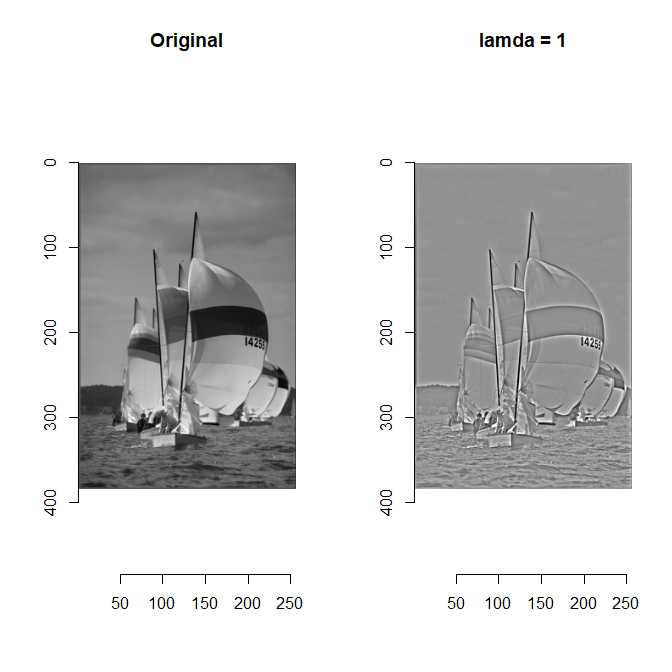# DCT denoising

DCT denoising is a simple and effective denoising algorithm using local DCT thresholding.

## the parameter sdn

sdn is a standrd deviation of noise of image.

The noise is strongly denoised if sdn is large.

## the parameter flag_dct16x16

flag_dct16x16 determiens window size of local patches. DCT denoising uses 8x8 windows or 16x16 window.

Larger window size does not bring significant improvement when noise level is low.

Larger window size outperforms significantly smaller window size when noise level is low.

noisy <- g + imnoise(dim = dim(g), sd = 0.1)
layout(matrix(c(1,3,2,4), 2, 2))
plot(g, main = "Original")
plot(noisy, main = "Noisy Boats")
DenoiseDCT(noisy, 0.1) %>% plot(., main = "Denoised (8x8 window)")
DenoiseDCT(noisy, 0.1, flag_dct16x16 = TRUE) %>% plot(., main = "Denoised (16x16 window)")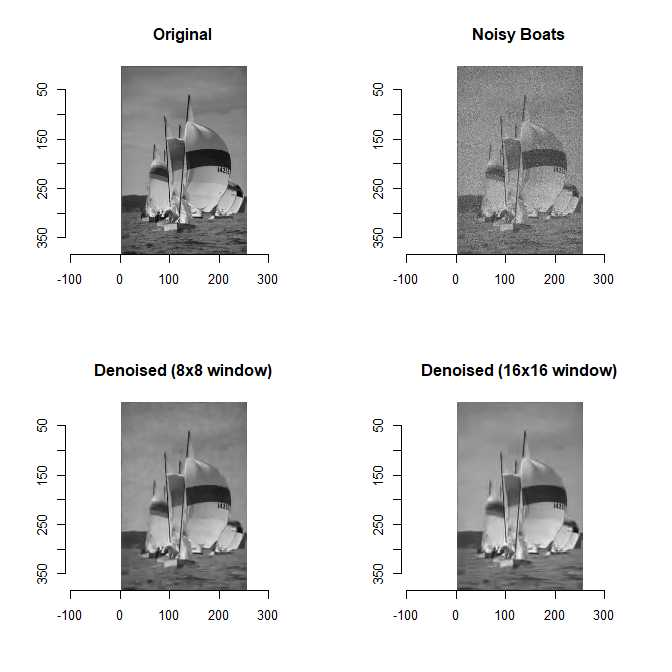# Iterative Triclass Thresholding

Iterative triclass thresholding is an iterative thresholding technique.

We need to set a rule to stop iteration.

We have two options. * set preset threshold (stopval) * set repeat number (repeatnum)

See [the intoroduction]https://shotaochi.github.io/2018/06/30/Iterative-Triclass-Thresholding.html) if you want to know about iterative triclass thresholding.

## the parameter stopval and repeatnum

stopval determines a preset threshold to stop iteration.

repeatnum determines how many times we repeat.

g <- grayscale(dogs)
layout(matrix(1:4, 2, 2, byrow = TRUE))
plot(g, main = "Original", axes=F)
ThresholdTriclass(g, stopval = 0.01) %>% plot(main = "stopval = 0.01", axes=F)
ThresholdTriclass(g, repeatnum = 1) %>% plot(main = "repeatnum = 1", axes=F)
ThresholdTriclass(g, repeatnum = 3) %>% plot(main = "repeatnum = 3", axes=F)